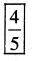# PSEB 5th Class Maths Solutions Chapter 4 Fractions Intext Questions

Punjab State Board PSEB 5th Class Maths Book Solutions Chapter 4 Fractions InText Questions and Answers.

## PSEB 5th Class Maths Solutions Chapter 4 Fractions InText Questions

Try These : (Textbook Page No.86)

Question 1.
Write the fraction of coloured stars.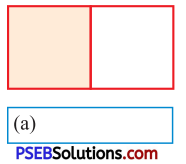Solution:
$$\frac{1}{2}$$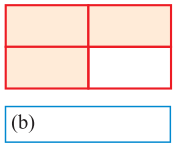Solution:
$$\frac{3}{4}$$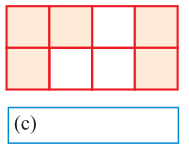Solution:
$$\frac{5}{8}$$Question 2.
Colour the diagram according to given fraction :

(a) $$\frac{2}{3}$$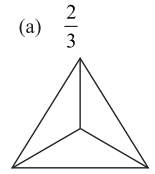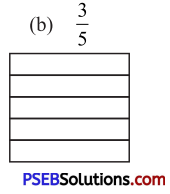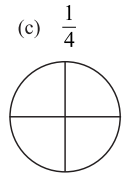Solution: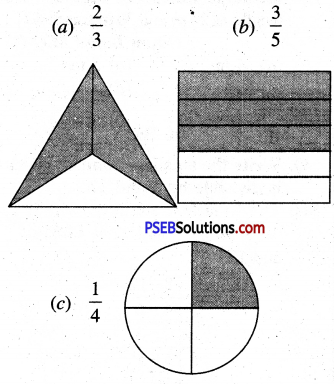Question 3.
In fraction $$\frac{2}{3}$$, numerator is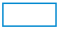and denominator is.
Solution:
In fraction $$\frac{2}{3}$$, numerator is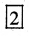and denominator is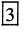.Question 4.
In fraction $$\frac{1}{2}$$, numerator isand denominator is.
Solution:
In fraction $$\frac{1}{2}$$, numerator is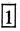and denominator is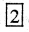.

Question 5.
Write the fraction with numerator 4 and denominator 5:Solution:
Write the fraction with numerator 4 and denominator 5: